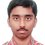Function Problem

This problem appeared in one of the exams which I appeared in. I am mentioning it here only because I could not solve it. Here goes the problem:

Let f(x) =$\frac{1}{x+2 cos(x)}$, x $\geq$0

Find the set A={y| y $\in$R, y=f(x)Note by Nishant Sharma
6 years, 5 months ago

This discussion board is a place to discuss our Daily Challenges and the math and science related to those challenges. Explanations are more than just a solution — they should explain the steps and thinking strategies that you used to obtain the solution. Comments should further the discussion of math and science.

When posting on Brilliant:

• Use the emojis to react to an explanation, whether you're congratulating a job well done , or just really confused .
• Ask specific questions about the challenge or the steps in somebody's explanation. Well-posed questions can add a lot to the discussion, but posting "I don't understand!" doesn't help anyone.
• Try to contribute something new to the discussion, whether it is an extension, generalization or other idea related to the challenge.
• Stay on topic — we're all here to learn more about math and science, not to hear about your favorite get-rich-quick scheme or current world events.

MarkdownAppears as
*italics* or _italics_ italics
**bold** or __bold__ bold

- bulleted
- list

• bulleted
• list

1. numbered
2. list

1. numbered
2. list
Note: you must add a full line of space before and after lists for them to show up correctly
paragraph 1

paragraph 2

paragraph 1

paragraph 2

> This is a quote
This is a quote
# I indented these lines
# 4 spaces, and now they show
# up as a code block.

print "hello world"
# I indented these lines
# 4 spaces, and now they show
# up as a code block.

print "hello world"
MathAppears as
Remember to wrap math in $$...$$ or $...$ to ensure proper formatting.
2 \times 3 $2 \times 3$
2^{34} $2^{34}$
a_{i-1} $a_{i-1}$
\frac{2}{3} $\frac{2}{3}$
\sqrt{2} $\sqrt{2}$
\sum_{i=1}^3 $\sum_{i=1}^3$
\sin \theta $\sin \theta$
\boxed{123} $\boxed{123}$

Sort by:

All they are asking for is the range of the given function, within the domain, right?

- 6 years, 5 months ago

Thank you all for your suggestions and solutions. Though I had solved the problem(in the exam in a clumsy manner) but didn't get the minimum as 0, which now I can see very easily. That was a silly mistake on my part.

- 6 years, 5 months ago

The minimum will never be zero.

- 6 years, 5 months ago

Yeah you are right. Actually I should have written it as approaching 0, since it is not attained at a finite value of x.

- 6 years, 5 months ago

A=[6/(5Pi-6sqrt{3)),0)

- 6 years, 5 months ago

just consider the function g(x)= x +2 cosx, now f(x)= 1/g(x), so if g(x1)> g(x2), then f(x1)< f(x2), now g(pi/6)=(pi/6+ sqrt3), g(5pi/6)= (5pi/6 + sqrt3), it is obvious g(pi/6)<g(5pi/6), therefore f(pi/6)>f(5pi/6)

- 6 years, 5 months ago

g(5pi/6)=(5pi/6-sqrt(3)) pls check the minus sign....

- 6 years, 5 months ago

hey....may...i ask a simple doubt....what's this ISI 2013 ? :|

- 6 years, 5 months ago

an exam for indian statistical institute

- 6 years, 5 months ago

- 6 years, 5 months ago

that's...ok...but..is it something like iit,nit,engineering college.....? i mean what kind of study..courses...? act. i dont know abt. this college...:)

- 6 years, 5 months ago

thanksss :)

- 6 years, 5 months ago

f(pi/6)= 6/(pi+ 6sqrt3, now you decide which is larger, 6/(5Pi+6sqrt{3), or 6/(pi+ 6sqrt3

- 6 years, 5 months ago

I think the problem is that sinx = sin(pi/6) has various solutions.You have simply taken the minimum value of x.Hence the wrong answer (verified using graphing calculator).Maximum is at x = 5pi/6

- 6 years, 5 months ago

its obvious as x tends to become larger, f(x) tends loser to 0, but never 0, so the infimum of the function is 0.

next step is to see that f(x), is continuous from 0 to infinity, so f(x), varies continously from its infimum to supremum, next step is to find the supremum, so we find the minima of the function x+ 2cosx., which occurs at x= pi/6, so maxima of f(x)= f(pi/3)= 6/(pi+ 6sqrt3), so A=( 0,6/(pi+ 6sqrt3) ].

- 6 years, 5 months ago

At pi/6 it reaches minimum not maximum. Only at 5pi/6 it reaches maximum plot the graph and see.

- 6 years, 5 months ago

At pi/6 x+2cos(x) reaches maximum therefore 1/(x+2cos(x)) reaches local minimum. but at 5pi/6 x+2cos(x) reaches minimum therefore 1/(x+2cos(x)) reaches maximum...

- 6 years, 5 months ago

pi/6 is untrue. if you differentiate f'(x) again and put x=pi/6. f''(x) at x=pi/6 is more than 0. So pi/6 is local minimum.

- 6 years, 5 months ago

Please plot the graph using a graphing utility and see

- 6 years, 5 months ago

check your graphs once more, or atleast try to see the proof that i just postd

- 6 years, 5 months ago

$x=\frac{\pi}{6}$ is the local minimum.

- 6 years, 5 months ago

Which would make x=5pi/6 maximum

- 6 years, 5 months ago